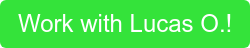Having worked through a long physics problem, you finally have an answer. How do you know if it’s right and all that work wasn’t for naught? In this post, I will cover a few quick strategies that can help rule out wrong answers.

We will use the following problem as an example:## Work through the problem with variables

One simple strategy that you can try from the very beginning of the problem is to work to the solution using only variables and not values given in the problem. There are several benefits to this strategy. The first is that it is easier to go back and check for algebra errors with variables as opposed to numbers. If an error has been made, this will make it easier to pinpoint and correct it. The second benefit is that computed values are much simpler to check because all numerical calculations are at the end. This means that the actual computation only needs to be verified at the end rather than throughout the problem. Additionally, because the numbers are all plugged in together at the end, rounding errors can be avoided because rounding is only done once rather than being distributed throughout the problem.## Order of magnitude

Once you have calculated your answer, you should consider whether your result is reasonable or not. You should try to get a sense of the values of different quantities in SI units. This can be challenging for Americans because we do not use the metric system but it is an important skill to develop. For instance, it’s helpful to know that if a baseball pitcher throws a ball at 1 m/s or 1000 m/s, these rates are probably unreasonably slow and fast, respectively. You may want to remember approximate conversions between SI and units you are more familiar with, if that is easier. For instance, a meter is approximately equal to a yard.## Significant Figures

While not a method for checking solutions, it is still important to remember to check your significant figures. Some tests remove points for the wrong number of significant figures, so be sure not to lose easy points!## Units

It is always important to include the units in the solution. It is typically easiest to solve problems using only SI units but there will be cases where this is not true. Whatever units you use, make sure that you are being consistent throughout the entire problem. For example, if you choose to use liters for volume instead of cubic meters, make sure you do so for every quantity in the problem. I typically list all the known values given by the problem at the top of my page where I am solving the problem. This is the point at which I convert to the units that I will be using to solve the problem so that I don’t forget later.## Dimensional Analysis

Dimensional analysis is one of the most powerful tools for checking an answer and relies on verifying that the units of the solution are correct. For example, we know that any solution for an acceleration must have units of m/s2 if SI units are used. Dimensional analysis highlights algebra errors because this typically causes the units not to work out correctly. To use this technique, all units should be converted to SI base units like kilograms, meters and seconds. For instance, a joule is equal to a kilogram meter squared per second squared.## Taking limits

An additional benefit to finding your final answer in terms of variables is that it allows you to take limits. You can check whether the solution makes sense by looking at the effects of increasing or decreasing terms in the solution. This requires some physical intuition and is a skill that you will build as you solve more problems. Useful limits tend to be 0 and infinity for most quantities. For angles, 0 and 90 degrees tend to be useful limits as they reduce cosine and sine to 0 or 1.# Final Thoughts

It is always important to make sure that the your answer to any physics problem is reasonable. Take advantage of these techniques to avoid and catch algebra and computational errors. Implementing an organized approach to problem solving will help you solve problems correctly with more consistency. With practice, these techniques will become second nature and will help you verify that your solution is the correct one.

Lucas received his BS from the Massachusetts Institute of Technology in Physics and is currently pursuing his PhD in Physics from Harvard University. Lucas’ interest in condensed matter physics began while he was an undergraduate, when he became an author on two published papers on graphene. He currently researches quantum computing in semiconductors and was awarded the Quantum Computing Graduate Fellowship.

Lucas has been a TA for physics courses at both MIT and Harvard, and was awarded the White Award for Excellence in Teaching in 2019. Additionally, he has over 600 hours of experience teaching online high school math and physics courses and has taught at a math summer camp for elementary through high school students. His teaching philosophy focuses on learning to ask the right questions, building intuition so that equations are more approachable, and breaking problems down into simpler parts. His goal is to help his students develop a physics mindset by emphasizing key concepts like conservation laws and symmetries. He believes that the best response to a question is another question that guides the student in the right direction so that the student has the benefit of discovering the answer for herself. He also stresses test-taking strategies that are often useful for physics problems.Want to learn more about physics? Take a look at some of our previous blog posts on the subject, below!

Tags: physics